# Research Vignette: Entangled Solutions to Logic Puzzles

The Fall 2016 Simons Institute program on Logical Structures and Computation focused on four themes: a) finite and algorithmic model theory; b) logic and probability; c) logic and quantum mechanics; and d) logic and databases. In this Research Vignette, I want to highlight one of the outcomes of this fruitful program in the form of an emerging direction of research that, quite surprisingly, touches on all four themes at once.

Two-player refereed games
A game is played by Alice and Bob, who confront a verifier. After an initial gathering to agree on a common strategy, Alice and Bob will not be allowed to communicate during the game. The game starts with the verifier privately choosing a pair of queries (p,q) ∈ P × Q according to some probability distribution π known to everyone; the verifier sends p to Alice and q to Bob. Alice and Bob reply with a pair of answers (r,s) ∈ R × S according to their previously agreed strategy; Alice replies r and Bob replies s. Upon receipt of the answers, the verifier accepts or rejects depending on whether a predicate V (p,q,r,s) also known to everyone holds or not. Alice’s and Bob’s goal is to maximize the probability of winning, i.e., making the verifier accept. Let this maximum be called the value of the game.

How could we model the value of this game? One perfectly natural approach would be to model it as a constraint-optimization problem. The possible queries are the variables, the possible answers are the values for these variables, and the verifier’s predicate V (p,q,r,s) defines the constraints, weighted by π(p,q). The optimal strategy would then be an assignment of values to variables that maximizes the total weight of satisfied constraints.

However, this model does not seem to capture all the different ways Alice and Bob could interact in their initial gathering. For example, Alice and Bob could have agreed to use the outcomes of a number of coin-flips, which they could have recorded in their respective memory sticks, to determine their answers during the game. As long as they don’t communicate during the game, this form of interaction is allowed! A better model for this situation would then seem to be that Alice and Bob choose functions of the form A : P × T R and B : Q × T S, where T denotes the set of potential coin-flip results, which has an associated probability distribution σ. Their goal would now be to maximize the average weight:

It turns out that in this particular case, there is no difference in the optimal value of the two models; but one could easily imagine that if we missed this one, we could well be missing other ways that Alice and Bob could interact in defining their strategy. And indeed, we are missing some such forms of interaction.

Suppose that Alice and Bob, when they design their strategy, agree to prepare a pair of elementary particles in a suitable entangled state, in the way they were taught was possible in their quantum mechanics class. When they separate, Alice takes one of the particles with her and Bob takes the other. When they play, Alice and Bob perform certain measurements on their respective particles depending on the query each one gets, and then they use the outcomes to determine their answers. Notice that Alice and Bob still stay separated from each other; they just perform local measurements on their local particles! The question is: could the fact that the particles were entangled in the past give Alice and Bob a strategy that has greater probability of winning?

Quantum vs. hidden-variable theories
The peculiar properties of quantum entanglement have fascinated physicists, mathematicians, logicians, and philosophers since the 1920’s. It is well-known that such giants as Einstein were initially reluctant to accept the uncertainty principle of quantum mechanics in its full strength. Others, such as von Neumann, were profoundly inspired by the topic to develop their deepest work. In more recent times, theoretical computer scientists have brought forth a computational and information-theoretic perspective that, hopefully, sheds some more light. The origins of this approach go back to the famous 1964 article of J.S. Bell, “On the Einstein-Podolsky-Rosen Paradox.”

Following Bell, let’s consider a Gedankenexperiment. Before the experiment starts, a large number N of pairs of particles of so-called spinare independently prepared in an entangled state, the singlet state. The experiment starts when Alice and Bob travel to distant planets with one particle each from each pair. Once in place, they measure the spins of their particles along different axes. Alice uses axis a and Bob uses axis b, where a and b are 3-dimensional unit vectors chosen by the verifier. As they do their measurements, they collect outcomes r1,,rN ∈{+1,−1} for Alice, and s1,,sN ∈{+1,−1} for Bob, which they send back to the verifier. At this point Bell asked: what are we to expect for the value of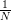i=1Ns iri? In other words, if the verifier counts the number of times that ri = si and subtracts the number of times that ri ≠ si, what are we to expect?

Bell computed what quantum theory predicts for these questions, and he concluded that one is to expect that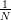i=1Nr isi approaches − cos θ as N grows, where θ ∈  is the angle between the axis vectors a and b. Put differently, if we ask the verifier to accept any single pair of data (ri,si) only if ri = si, one is to expect that the verifier would accept with probability approachingcos θ. The point of Bell’s study was to contrast this calculation with what would be expected from a local hidden-variable theory of the type Einstein and his co-authors had hoped would explain the uncertainty in the experiment. In such a model, Bell argued, the statistics ofi=1Nr isi would have to take the form of an average ∑ tT σ(t)i=1Nr i(t)si(t), and he went on to prove that in such a case it would have to approach the quantity −1 +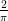θ. In other words, a verifier that accepts only if ri = si would have to accept with probability approaching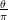. The discrepancy between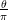and the earliercos θ is particularly noticeable at θ ≈ 133.56 where the ratio is minimized and is as small as 0.878567.

The analysis in Bell’s Theorem can be turned into a game in which entangled strategies give higher probability of winning than unentangled ones. The question arises, however, of whether this difference can be witnessed only statistically, i.e., quantitatively, or if it manifests itself in more absolute terms, i.e., qualitatively. In other words, could there be a game in which Alice and Bob can make the verifier accept with certainty if they use entanglement but not if they don’t? Such questions had also been considered by physicists, including Bell himself, with the conclusion that, also at this level, entangled strategies are superior to unentangled ones. This type of refinement of the sense in which entanglement is more powerful, which led Mermin to coin Kell-Kochen-Specker type theorems in his beautiful paper, “Hidden variables and the two theorems of John Bell,” ties better to the problems of interest to logicians, and will take our attention for the rest of this Vignette.

Quantum relaxations of constraint satisfaction problems
Take an instance of the constraint satisfaction problem with Boolean variables X1,,Xn ranging over {+1,−1}, and constraints given by, say, polynomial equations of the form P(Xi1,,Xik) = 0. There is a well-known game that models this problem: the verifier chooses both a constraint and a variable that appears in this constraint, sends the constraint to Alice and the variable to Bob, and expects an assignment that satisfies the constraint from Alice, and an assignment that agrees with Alice’s on the pointed variable from Bob. It is easy to see that the solutions to the constraint-satisfaction instance give rise to (unentangled) strategies that win with certainty in the game, and vice-versa. What about entangled strategies? What do they correspond to at the level of the constraint-satisfaction instance? In their ICALP 2014 paper, theoretical computer scientists Richard Cleve and Rajat Mittal addressed this question and, to answer it, introduced the following quantum relaxation of the constraint-satisfaction problem:

Let the variables X1,,Xn range over Hermitian d-dimensional matrices in ℂd×d for some arbitrary but unspecified dimension d ≥ 1. Impose the conditions that Xi2 = I, where I is the identity matrix, and that the matrix product on Xi and Xj commutes whenever Xi and Xj appear together in at least one of the given polynomial equations. Note that the case d = 1 is no relaxation at all since the 1-dimensional matrices are just scalars, whose product obviously commutes, and the equations Xi2 = I translate to X i ∈{+1,−1}. Cleve and Mittal proved that entangled winning strategies in the game give rise to solutions to this relaxed version of the problem, and vice-versa.

Now we can ask the type of questions that Bell asked. Could there be a system of polynomial equations that is quantum-satisfiable in the sense above but not classically satisfiable? Some well-known such examples come from the work of the physicists in their proofs of the Bell-Kochen-Specker Theorems; the best known is the Mermin-Peres Magic Square made of six polynomial equations (the three rows and the three columns in the square below) over nine variables:

Seen as a logic puzzle of the kind that one used to find in newspapers, this appears to have no solution: all variables appear exactly twice so the left-hand sides of the equations multiplied together give 1, while the right-hand sides give −1. In contrast, the system is quantum-satisfiable by means of a 4-dimensional solution made of suitable Kronecker products of the classical Pauli matrices (Fig 3. in Mermin’s paper — see Notes for further reading, below).

Emerging directions
In his series of lectures in the Logic and Computation Boot Camp that took place at the begining of the program, Samson Abramsky gave an introduction to the topic of Bell-type results from a logician’s viewpoint. A recurrent theme within the lectures was the extent to which local constraints on a system, such as the outcomes of physical measurements, may or may not influence its global properties. Put this generally, this is indeed a common theme of most subareas of logic in computer science. It appeared in Panangaden’s lectures on the analysis of probabilistic systems while constructing continuous probability spaces that meet the local constraints that arise from conditioning. It also appeared in Kolaitis’ lectures on database theory while discussing how the local structure of a conjunctive query can influence the size of its output and the computational complexity of computing it. And it appeared in Dawar’s course on finite and algorithmic model theory as the fundamental question of whether the local structure of a finite model could determine it up to isomorphism. I will close this Vignette with a discussion on a research question that was raised at the program, which links this last topic with Bell-Kochen-Specker-type theorems, and which was successfully answered in a team-effort during the program.

The work of Cleve and Mittal referred to above is the continuation of a recent trend that takes classical combinatorial problems and relaxes them into quantum analogues: quantum chromatic numbers, quantum homomorphisms, quantum Lovász theta, etc. Let us take quantum graph isomorphism. We are given two graphs G and H. The verifier chooses two vertices from either graph and sends them to Alice and Bob, one vertex each. If Alice gets a vertex u from G, she must reply with a vertex v from H, and vice-versa. If Bob gets a vertex u′ from G, he must reply with a vertex v′ from H, and vice-versa. The verifier will accept if and only if the two pointed vertices from G satisfy the same equalities and edge relationships as the two pointed vertices from H; i.e., he will accept precisely when u = u′ if and only if v = v′, and u Gu′ if and only if v Hv′. It is not hard to see that Alice and Bob have an unentangled strategy that wins this game with certainty if and only if G and H are isomorphic. Are there pairs of graphs on which Alice and Bob win with entanglement but not without?

The graph isomorphism problem is of course of fundamental importance in theoretical computer science. For one thing, it is one of the few problems in the complexity class NP that is not known to be polynomial-time solvable or NP-complete. Thanks to the recent breakthough of Babai, we now know that the problem is solvable in quasipolynomial time, and thanks to the earlier work in probabilistic proof systems, we already had strong evidence that the problem cannot be NP-complete. These two facts could explain why it has always been so hard to find hard-to-solve instances of the graph isomorphism problem. To put it graphically, if you give me a reasonably smart heuristic to solve the graph isomorphism problem, chances are that I will have a hard time finding an example on which your heuristic fails. For this very same reason, finding two graphs on which Alice and Bob can win the isomorphism game with entanglement but not without does not look like a very easy question.

It turns out that similar questions had been asked previously in descriptive complexity theory in the 1980’s. The fundamental problem of that area, which has its origins in the theory of relational databases, is whether there is a logic that is able to express all and only the isomorphism-invariant graph properties that can be decided in polynomial time. In the 1980’s some candidate logics were put forward, until one was found to resist all attempts at a counterexample. This was the logic now called Fixed-Point Logic with Counting (FPC). Eventually, in a breakthrough that became known as the CFI-construction, J. Y. Cai, M. Fürer and N. Immerman found a counterexample: they proved that the isomorphism problem for graphs with color-classes of bounded size, which is a special case that is solvable in polynomial time, cannot be expressed in FPC.

Besides its original purpose, the CFI-construction became also the canonical “hard-to-solve” instance for combinatorial heuristics for the graph isomorphism problem. For one thing, it was the first construction to show that there exist pairs of non-isomorphic graphs for which the classical vertex-refinement heuristic, when extended to tuple-refinement in the straightforward way, would need to use tuples of unbounded length before it detects that the graphs are not isomorphic. Could the same construction be used to solve our problem of finding non-isomorphic graphs that are nonetheless quantum isomorphic? One difficulty in going this way is that nobody knows of a systematic method to decide if two given graphs are quantum isomorphic; as far as we know, the problem could be even undecidable. For this reason, it would have been more natural to start by constructing two graphs that are quantum isomorphic by design, and only then show that they are not classically isomorphic. But CFI was already there, and we had to give it a try. So we tried it, and… it worked!

The key to finding the solution was the previously noted fact that, in the context of the logical and universal-algebraic approaches to the Feder-Vardi Dichotomy Conjecture for constraint satisfaction problems, the CFI-construction can be interpreted as a system of parity equations in which each variable appears in exactly two equations. Coincidentally (or not), these are precisely the type of systems of equations that underly the Mermin-Peres Magic Square and similar constructions. From there, a few calculations sufficed to realize that Mermin’s solution to the magic square could be reused, and the problem was solved.

Bell’s paper appears in Physics 1, 1964: 195-200. The ratio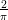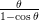in our exposition of Bell’s analysis is exactly the same as Goemans-Williamson’s approximation ratio for MAX-CUT and is related to Grothendieck’s constant; this is hardly a coincidence. See for example the paper by Regev and Vidick, “Quantum XOR Games,” ACM TOCT, 7(4), 2015, n. 15, and references therein. For the Bell-Kochen-Specker Theorem and the magic square see Mermin’s Rev. Mod. Phy. 65(3), 1993. Cleve and Mittal’s paper appeared in the Proceedings of ICALP 2014 and also QIP 2014 and arXiv:1209.2729 [quant-ph]. The Cai-Fürer-Immerman paper appears in Combinatorica 12(4), 1992: 389-410, with an earlier version in FOCS 1989. The new results on quantum graph isomorphism appear in Atserias, Mančinska, Roberson, Šámal, Severini, and Varvitsiotis, “Quantum and non-signalling graph isomorphisms,” arXiv:1611.09837 [quant-ph], with a shorter version in ICALP 2017. Two other recent papers that report work along these lines are Atserias, Kolaitis and Severini, “Generalized Satisfiability Problems via Operator Assignments,” arXiv:1704.01736 [cs.LO]; and Abramsky, Dawar and Wang, “The pebbling comonad in finite model theory,” arXiv:1704.05124 [cs.LO]. This last will appear in shorter form in LICS 2017.